## Error Detection in Computer Networks-

 Error detection is a technique that is used to check if any error occurred in the data during the transmission.

Some popular error detection methods are-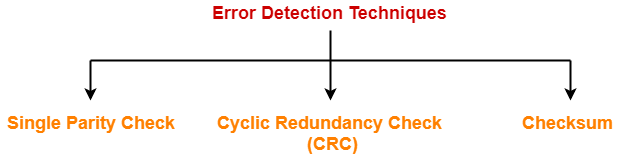1. Single Parity Check
2. Cyclic Redundancy Check (CRC)
3. Checksum

## Cyclic Redundancy Check-

• Cyclic Redundancy Check (CRC) is an error detection method.
• It is based on binary division.

## CRC Generator-

• CRC generator is an algebraic polynomial represented as a bit pattern.
• Bit pattern is obtained from the CRC generator using the following rule-

 The power of each term gives the position of the bit and the coefficient gives the value of the bit.

## Example-

Consider the CRC generator is x7 + x6 + x4 + x3 + x + 1.

The corresponding binary pattern is obtained as-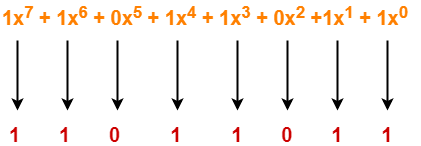Thus, for the given CRC generator, the corresponding binary pattern is 11011011.

## Properties Of CRC Generator-

The algebraic polynomial chosen as a CRC generator should have at least the following properties-

### Rule-01:

• It should not be divisible by x.
• This condition guarantees that all the burst errors of length equal to the length of polynomial are detected.

### Rule-02:

• It should be divisible by x+1.
• This condition guarantees that all the burst errors affecting an odd number of bits are detected.

## Important Notes-

If the CRC generator is chosen according to the above rules, then-

• CRC can detect all single-bit errors
• CRC can detect all double-bit errors provided the divisor contains at least three logic 1’s.
• CRC can detect any odd number of errors provided the divisor is a factor of x+1.
• CRC can detect all burst error of length less than the degree of the polynomial.
• CRC can detect most of the larger burst errors with a high probability.

## Steps Involved-

Error detection using CRC technique involves the following steps-

### Step-01: Calculation Of CRC At Sender Side-

At sender side,

• A string of n 0’s is appended to the data unit to be transmitted.
• Here, n is one less than the number of bits in CRC generator.
• Binary division is performed of the resultant string with the CRC generator.
• After division, the remainder so obtained is called as CRC.
• It may be noted that CRC also consists of n bits.

### Step-02: Appending CRC To Data Unit-

At sender side,

• The CRC is obtained after the binary division.
• The string of n 0’s appended to the data unit earlier is replaced by the CRC remainder.

• The newly formed code word (Original data + CRC) is transmitted to the receiver.

### Step-04: Checking at Receiver Side-

• The transmitted code word is received.
• The received code word is divided with the same CRC generator.
• On division, the remainder so obtained is checked.

The following two cases are possible-

### Case-01: Remainder = 0

If the remainder is zero,

• Receiver assumes that no error occurred in the data during the transmission.

### Case-02: Remainder ≠ 0

If the remainder is non-zero,

• Receiver assumes that some error occurred in the data during the transmission.

## Problem-01:

A bit stream 1101011011 is transmitted using the standard CRC method. The generator polynomial is x4+x+1. What is the actual bit string transmitted?

## Solution-

• The generator polynomial G(x) = x4 + x + 1 is encoded as 10011.
• Clearly, the generator polynomial consists of 5 bits.
• So, a string of 4 zeroes is appended to the bit stream to be transmitted.
• The resulting bit stream is 11010110110000.

Now, the binary division is performed as-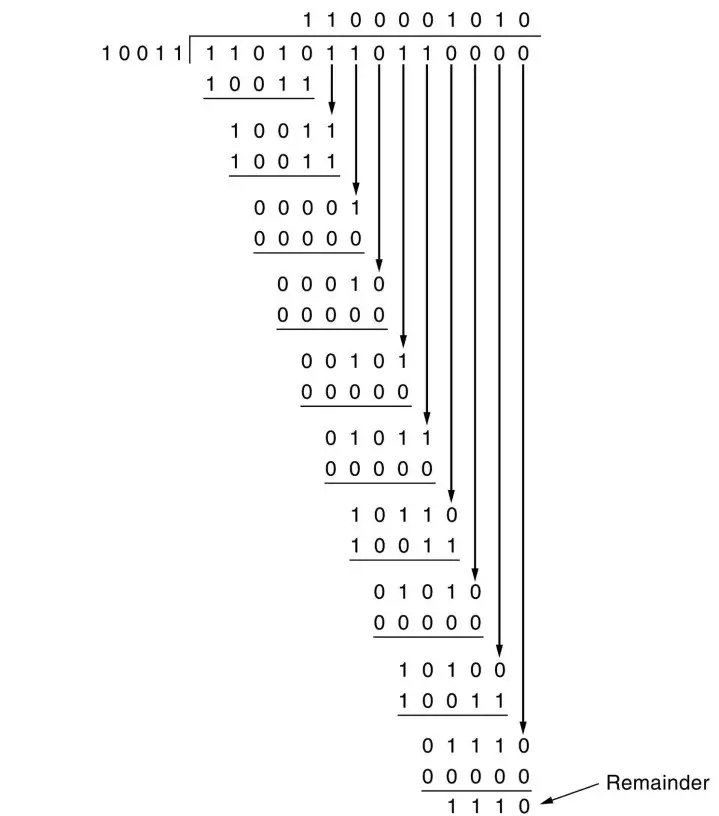From here, CRC = 1110.

Now,

• The code word to be transmitted is obtained by replacing the last 4 zeroes of 11010110110000 with the CRC.
• Thus, the code word transmitted to the receiver = 11010110111110.

## Problem-02:

A bit stream 10011101 is transmitted using the standard CRC method. The generator polynomial is x3+1.

1. What is the actual bit string transmitted?
2. Suppose the third bit from the left is inverted during transmission. How will receiver detect this error?

## Solution-

### Part-01:

• The generator polynomial G(x) = x3 + 1 is encoded as 1001.
• Clearly, the generator polynomial consists of 4 bits.
• So, a string of 3 zeroes is appended to the bit stream to be transmitted.
• The resulting bit stream is 10011101000.

Now, the binary division is performed as-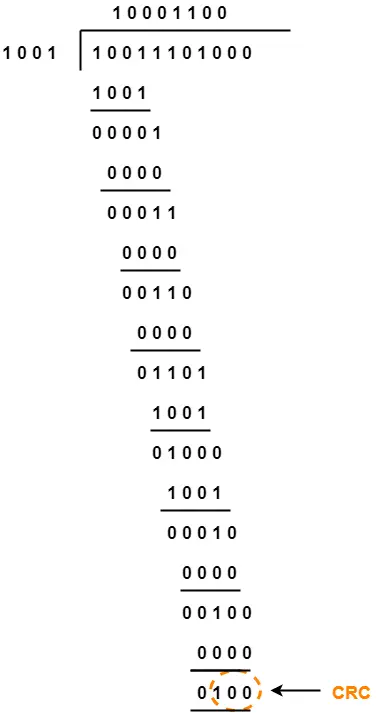From here, CRC = 100.

Now,

• The code word to be transmitted is obtained by replacing the last 3 zeroes of 10011101000 with the CRC.
• Thus, the code word transmitted to the receiver = 10011101100.

## Part-02:

According to the question,

• Third bit from the left gets inverted during transmission.

Now,

• Receiver performs the binary division with the same generator polynomial as-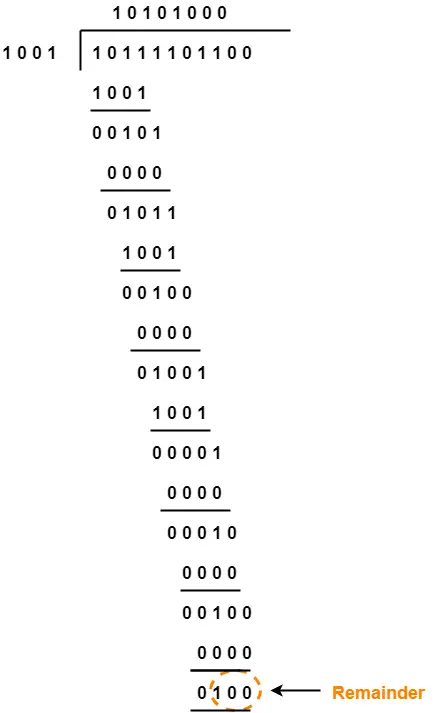From here,

• The remainder obtained on division is a non-zero value.
• This indicates to the receiver that an error occurred in the data during the transmission.
• Therefore, receiver rejects the data and asks the sender for retransmission.

To gain better understanding about Cyclic Redundancy Check,

Watch this Video Lecture

Next Article- Checksum

Get more notes and other study material of Computer Networks.

Watch video lectures by visiting our YouTube channel LearnVidFun.